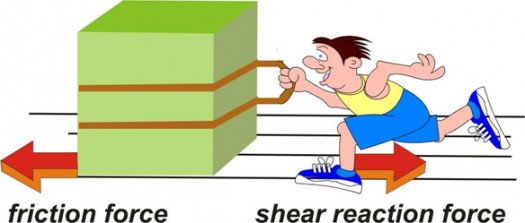# Quiz: Types Of Friction! Trivia Knowledge Test

10 Questions | Attempts: 273
ShareSettingsDid you know that there is more than one type of friction? Do you know the laws that regulate all these types of frictions and their most basic differences? Friction is normally defined as the force resisting the relative motion of solid surfaces or fluid layers. So, what do you think you know about it? Take our quiz and find out now.

• 1.
What is dry friction?
• A.

It's a force that deletes the relative lateral motion of 2 solid surfaces in contact

• B.

It's a force that depletes the relative lateral motion of 2 solid surfaces in contact

• C.

It's a force that opposes the relative lateral motion of 2 solid surfaces in contact

• D.

It's a force that raises the relative lateral motion of 2 solid surfaces in contact

• 2.
What is fluid friction?
• A.

It describes the friction  between layers of a viscous fluid that are moving relative to each other

• B.

It describes the friction  between a fluid that is moving

• C.

It describes the friction  between layers of a heavy fluid that are moving relative to each other

• D.

It describes the friction  between layers of a oily fluid that are moving relative to each other

• 3.
What is lubricated friction?
• A.

It's a case of solid friction where a lubricant fluid separates 2 solid surfaces

• B.

It's a case of fluid friction where a lubricant fluid separates 2 solid surfaces

• C.

It's a case of semi solid friction where a lubricant fluid separates 2 solid surfaces

• D.

It's a case of semi fluid friction where a lubricant fluid separates 2 solid surfaces

• 4.
What's skin friction?
• A.

It's a component of drag of a fluid across the surface of a body

• B.

It's a component of drag, the force resisting the motion of a fluid across the surface of a body

• C.

It's a component of drag, the force resisting a fluid across the surface of a body

• D.

It's a component of the force resisting the motion of a fluid across the surface of a body

• 5.
What is internal friction?
• A.

It's the force resisting motion between the elements making up a solid material while it undergoes deformation

• B.

It's the force resisting motion between the elements making up a solid material

• C.

It's the force resisting motion while it undergoes deformation

• D.

It's the force between the elements making up a solid material while it undergoes deformation

• 6.
How many types of frictions are there?
• A.

5

• B.

4

• C.

3

• D.

7

• 7.
What happens when surfaces in contact move relative to each other?
• A.

The friction between the 3 surfaces converts kinetic energy into thermal energy

• B.

The friction between the 2 surfaces converts energy into thermal energy

• C.

The friction between the 2 surfaces converts solid energy into thermal energy

• D.

The friction between the 2 surfaces converts kinetic energy into thermal energy

• 8.
What does it mean when friction between 2 kinetic energy into thermal energy?
• A.

That it converts heat into work

• B.

That it converts fire to heat

• C.

That it converts work to heat

• D.

That it converts heat to fire

• 9.
Why is friction important for land vehicles?
• A.

Because they rely on friction for acceleration, deceleration, and changing direction

• B.

Because they rely on friction for changing direction

• C.

Because they rely on friction for acceleration

• D.

Because they rely on friction for deceleration, and changing direction

• 10.
What causes dry friction?
• A.

A combination of inter-surface adhesion and surface roughness

• B.

A combination of surface roughness, and surface contamination

• C.

A combination of inter-surface adhesion, surface roughness, surface deformation, and surface contamination

• D.

A combination of surface deformation and surface contamination

## Related TopicsBack to top
×

Wait!
Here's an interesting quiz for you.